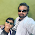## Friday, January 11, 2013

### Common HPLC Calculations:

Capacity Factor / Retention Factor / Capacity Ratio:  k1 (K Prime)

k1 = T(R) - T(0) / T(0)
where T(R) equals the retention time of the peak in minutes and T(0) is
the retention time of an unretained peak. *For chromatography to take place, K Prime must be > 1.00 and for most modes of chromatography, should be greater than 1.5 or 2.0 for all samples !

Tailing Factor: USP: 't'

t = W(5.0)/tw/2

where tw equals the distance between peak front and T(R) at 5% of peak height units. W(5.0) equals peak width at 5% height, in minutes.

Theoretical Plates: USP and ASTM, 'N'

N = 5.54 x (T(R)/W(50))2

Assumes width at peak half height (50)

Resolution: USP, ASTM, 'R'

R = (T(R)(b)-T(R)(a)) x 2.35/(W(50)(b) + W(50)(a))/2

Assumes width at half height (50%) with peaks (a) and (b).

*Notes: Visually, "Baseline" resolution is R = 1.5. Your goal should be R = or > 2.0. ** R of 1.5 provides 99.8% separation which means you cannot accurately quantify a 0.1% impurity so develop the method to have a resolution value of at least 2.0.

Note: The appropriate formula(s) for use with your samples may depend on which of the many pharmaceutical guidelines and regulations apply in your country. Always consult the appropriate guidelines.

1.2.3.